The mole

just like a DOZEN, only bigger

Solutions

 Dozen number multiply by 12 Mole number multiply by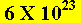1 1 X 12 = 12 1 1 X=3 3 X 12 = 36 3 3 X=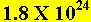4 4 X 12 = 48 4 4 X=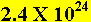5 5 X 12 = 60 5 5 X=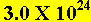2.5 2.5 X 12 = 15 2.5 2.5 X=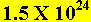3.5 3.5 X 12 = 42 3.5 3.5 X=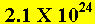10 10 X 12 = 120 10 10 X=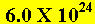0.1 0.1 X 12 = 1.2 0.1 0.1 X=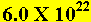0.2 0.2 X 12 = 2.4 0.2 0.2 X=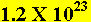Back to the exercises

Home